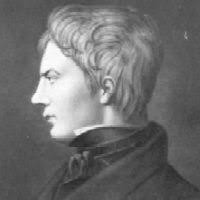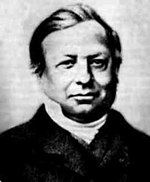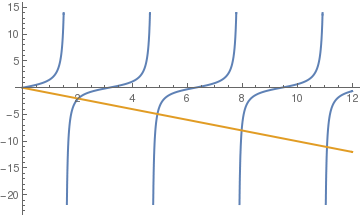# Preface

Sturm--Liouville theory is actually a generalization for infinite dimensional case the famous eigenvalue/eigenvector problems for finite square matrices that we discussed in Part I of this tutorial. Although a Sturm--Liouville problem can be formulated in operator form as L[ y ] = λy similarly to matrix eigenvalue problem Ax = λx, the operator L is usually an unbounded differential operator and y is a smooth function.

The corresponding theory that is known as Sturm--Liouville theory originated in two articles by the French mathematician of German decent Jacques Charles François Sturm (1803--1855).
1. Sturm, J.C.F., "Mémoire sur les Équations différentielles linéaires du second ordre", Journal de Mathématiques Pures et Appliquées, 1836, Vol. 1, pp. 106-186. [sept. 28, 1833]
2. Sturm, , J.C.F., "Mémoire sur une classe des d'Équations à différences partielles". Journal de Mathématiques Pures et Appliquées, 1836, Vol. 1, pp. 373--444.
Following the same 1836 year, Sturm together with his friend, the French mathematician Joseph Liouville (1809--1882), published very influential articles that provided crucial groundwork for the theory.
1. Sturm & Liouville, "Démonstration d'un théorème de M. Cauchy relatif aux racines imaginaires des équations". Journal de Mathématiques Pures et Appliquées, 1836, Vol. 1, pp. 278--289.
2. Sturm & Liouville, "Note sur un théorème de M. Cauchy relatif aux racines des équations simultanées", Comptes rendus de l'Académie des Sciences (English: Proceedings of the Academy of Sciences), 1837, Vol. 4, pp. 720--739.
3. Sturm & Liouville, "Extrait d'un Mémoire sur le développement des fonctions en séries dont les différents termes sont assujettis à satisfaire à une même équation différentielle lindaire, contenant un paramètre variable", Journal de Mathématiques Pures et Appliquées, 1837, Vol 2, pp. 220--233.
Comptes rendus de l'Académie des Sciences ((English: Proceedings of the Academy of sciences), 1837, Vol. 4, pp. 675--677.

Introduction to Linear Algebra with Mathematica

# Sturm--Liouville ProblemsJacques Sturm A classical Sturm–Liouville theory, named after Jacques Charles François Sturm (1803--1855) and Joseph Liouville (1809--1882), is the theory of a real second-order homogeneous linear self-adjoint differential equations with a parameter λ of the form
$\frac{\text d}{{\text d}x} \left[ p(x)\,\frac{{\text d}y}{{\text d}x} \right] - q(x)\, y + \lambda \,w(x)\,y(x) =0 , \qquad 0 < x < \ell ,$
subject to some homogeneous boundary conditions
$\alpha_1 y(0) - \alpha_2 y'(0) =0 , \qquad \beta_1 y(\ell ) + \beta_2 y' (\ell ) =0 , \qquad |\alpha_1 | + |\alpha_2 | \ne 0 \quad\mbox{and} \quad |\beta_1 | + |\beta_2 | \ne 0.$
Here y is a nontrivial (meaning not identically zero) function of the free variable x, and the functions p(x), q(x), and w(x) > 0 are specified at the outset. In the simplest of cases all coefficients are continuous on the finite closed interval [a,b], and p has continuous derivative. In this simplest of all cases, this nontrivial function y is called a solution if it is continuously differentiable on (a,b) and satisfies the given equation at every point in (a,b). The function w(x) is called the "weight" or "density" function.

Upon introducing the linear differential operator

$L\left[ x, \texttt{D} \right] = -\texttt{D}\,p(x)\,\texttt{D} + q(x) , \qquad \texttt{D} = \frac{\text d}{{\text d}x} ,$
and boundary operators
$B_0 y = \alpha_1 y(0) - \alpha_2 y'(0) \qquad\mbox{and}\qquad B_{\ell} y = \beta_1 y(\ell ) + \beta_2 y' (\ell ) ,$
we can rewrite the above problem as
$L\left[ x, \texttt{D} \right] y = w\,\lambda y , \qquad B_0 y = 0, \quad B_{\ell} y = 0.$
This boundary value problem has an obvious solution---identically zero function. Since we are not after such a trivial solution, we need something more. The Sturm--Liouville problem (S-L, for short) consists of two parts: the first part is about finding such values of parameter λ for which the problem has a nontrivial solution (not identically zero); such values are called eigenvalues. The second part includes determination of nontrivial solutions that are called eigenfunctions. Note that a Sturm--Liouville problem may have other constraints, not necessarily formulated above.

There are two kinds of Sturm--Liouville problems. One is called classical or regular when p(x) > 0 and w(x) > 0 for all points from the closed interval x∈[0,ℓ]. These assumptions are necessary to render the theory as simple as possible while retaining considerable generality. It turns out that these conditions are valid in many problems that we will consider shortly. If p(0) = 0, or p() = 0, or p(0) = 0 = p(), the Sturm--Liouville problem is said to be singular.Joseph Liouville Jacques Charles François Sturm (1803--1855) was a French mathematician of Switzerland descend. Charles spent his adult life in Paris. His primary interests were fluid mechanics and differential equations. Sturm with the Swiss engineer Daniel Colladon was the first to accurately determine the speed of sound in water. In mathematics, he won the coveted Grand prix des Scienes Mathematiques for his work in differential equations. Sturm held the chair of mechanics at the Sorbonne and was elected a member of the French Academy of Sciences. The asteroid 31043 Sturm is named for him. Sturm's name is one of the 72 names engraved at the Eiffel Tower.

Joseph Liouville was a French mathematician known for his work in analysis, differential geometry, and number theory and for his discovery of transcendental numbers---i.e., numbers that are not the roots of algebraic equations having rational coefficients. He was also influential as a journal editor and teacher. Joseph founded the Journal de Mathématiques Pures et Appliquées which retains its high reputation up to today.

In physics and other applications, many problems arise in the form of boundary value problems involving second order ordinary differential equations, written in self-adjoint form:

$L\left[ x, \texttt{D} \right] = \texttt{D} \left( p(x)\,\texttt{D} \right) + q(x) \qquad \mbox{or}\qquad L\left[ x, \texttt{D} \right] y = \frac{\text d}{{\text d}x} \left( p(x)\,\frac{{\text d}y}{{\text d}x}\right) + q(x) \, y ,$
where $$\texttt{D} = {\text d}/{\text d}x$$ is the derivative operator. Sturm was the first who generalized the well known matrix problem for eigenvalues/eigenvectors to the linear differential operators by considering the eigenvalue problem:
$L\left[ x, \texttt{D} \right] y + \lambda\,\rho (x)\, y =0$
for functions y(x) subject to some boundary conditions. Now this problem is referred to as the Sturm--Liouville problem. Here p(x), q(x), and ρ(x) > 0 are specified at the outset, which is usually is some interval (finite or not) of real axis ℝ.
Theorem: Properties of the regular Sturm--Liouville problem.
1. There exists an infinite number of real eigenvalues that can be arranged in increasing order λ1 < λ2 < λ3 < … λn < … such that λn →∞ as n→∞.
2. For each eigenvalue there is only one eigenfunction (up until nonzero multiple).
3. Eigenfunctions corresponding to different eigenvalues are linearly independent.
4. The set of eigenfunctions { yn }n≥0 corresponding to the set of eigenvalues is orthogonal with respect to the weight function w(x) on the interval x∈ [0, ℓ]:
$\left\langle y_n , y_k \right\rangle = \int_0^{\ell} y_n (x)\,y_k (x)\,w(x)\,{\text d}x = 0 \qquad \mbox{for} \quad n\ne k.$

The Fourier expansion of a square integrable generic function f ∈ 𝔏²[𝑎,b] on the interval (𝑎 , b) in terms of an orthogonal system of real-valued functions { φk } in 𝔏² is given by

$f(x) = \sum_{k\ge 1} c_k \varphi_k (x) ,$
where the coefficients are given by the inner product
$c_k = \left\langle \varphi_k , f \right\rangle = \int_a^b \varphi_k (x) \, f(x) \,{\text d}x , \qquad k=1,2,\ldots .$
These coefficients satisfy the so-called Bessel inequality:
$\sum_{k\ge 1} \left\vert c_k \right\vert^2 \le \left\langle f , f \right\rangle .$
A set of orthogonal functions { φk } in 𝔏² is called complete in the closed interval [𝑎,b] if for every function from 𝔏² the Parseval identity holds:
$\sum_{k\ge 1} \left\vert c_k \right\vert^2 = \left\langle f, f \right\rangle = \int_a^b \left\vert f(x) \right\vert^2 {\text d}x = \| f(x) \|^2 .$
The term complete was introduced by the famous Russian mathematician Vladimir Steklov (1864--1926). The above identity was stated by the famous French mathematician Marc-Antoine Parseval (1755--1836) in 1799.

The main reason to study Sturn--Liouville problems is that their eigenfunctions provide a basis for expansion for certain class of functions. This tells us that basis functions have close relationships with linear operators, and this basis is orthogonal for self-adjoint second order differential operators. In other words, solutions of differential equations can be approximated more efficiently by means of better basis functions. For example, a periodic function or solution is expressed more efficiently by periodic basis functions than by polynomials like the Taylor series.

For example, the linear operator $$L\left[ y \right] = y'' + \lambda \,y$$ determines the basis functions. When λ < 0, the basis functions will be

$\left\{ t^m e^{-n \sqrt{|\lambda |}\, t} , \ t^m e^{n \sqrt{|\lambda |}\, t} \right\}, \qquad m > 0, \quad n\ge 1.$
When λ = 0, it is represented by the set of basis functions
$\left\{ t^{2n} \ | \ n \ge 0\right\} .$
When λ > 0, the basis functions will be
$\left\{ t^m \cos \left( n\sqrt{\lambda}\,x \right) , \ t^m \sin \left( n\sqrt{\lambda}\,x \right) \right\}, \qquad m ≥ 0, \quad n\ge 1.$
Therefore, we expect that the basis of periodic functions
$\left\{ \cos \left( n\pi\,x \right) , \ \sin \left( n\pi\,x \right) \right\}, \qquad n\ge 0$
is more suitable for approximation of periodic functions than Taylor's series.

Example 1: Consider the Sturm--Liouville problem

$y'' + \lambda \,y =0 , \qquad y(0) = y(T) .$
Solutions of the differential equation $$y'' + \lambda \,y =0$$ depend on the sign of parameter λ. If λ = -μ2 is negative, the equation has two exponential linearly independent solutions $$y_1 = e^{\mu x} \quad\mbox{and}\quad y_2 = e^{-\mu x}$$ that also sometimes can be written through hyperbolic sine and cosine functions. These functions cannot be periodic, so negative λ is not an eigenvalue.

If λ = 0, the general solution of the differential equation $$y'' =0$$ is a linear function $$y = a + b\,x ,$$ which could be periodic only when b = 0. So a zero is an eigenvalue to which corresponds an eigenfunction---a constant function.

If λ = μ2 is positive, then the general solution becomes

$y = a\,\cos (\mu x) + b\,\sin (\mu x) ,$
with some constants 𝑎 and b. This function is periodic with period T if and only if μ is proportional to 2π/T; so we get eigenvalues
$\mu_n = \frac{2n\pi}{T} \qquad \Longrightarrow \qquad \lambda_n = \left( \frac{2n\pi}{T} \right)^2 , \quad n= 1,2,3,\ldots .$
The corresponding eigenfunctions are
$\cos \frac{2n\pi x}{T} \qquad\mbox{and} \qquad \sin \frac{2n\pi x}{T} , \quad n=1,2,3,\ldots .$
■

Example 2: Consider the Sturm--Liouville problem

$y'' + \lambda \,y =0 , \qquad y(0) = 0, \quad y(\ell ) =0 .$
When a function is specified at the boundary, then the corresponding boundary conditions are called after G. Dirichlet. Solutions of the differential equation $$y'' + \lambda \,y =0$$ depend on the sign of parameter λ. If λ = -μ2 is negative, the equation has two exponential linearly independent solutions $$y_1 = e^{\mu x} \quad\mbox{and}\quad y_2 = e^{-\mu x} .$$ Then the general solution becomes
$y(x) = c_1 e^{\mu x} + c_2 e^{-\mu x} ,$
with some constants c1 and c2. From the first boundary condition, we have
$y(0) = c_1 + c_2 =0 \qquad \Longrightarrow \qquad c_1 = - c_2 .$
The boundary condition at x = ℓ dictates
$y(\ell ) = c_1 \left( e^{\mu \ell} - e^{-\mu \ell} \right) = 2\,c_1 \sinh (\mu \ell) =0 .$
Since sine hyperbolic can be zero only at the origin, we conclude that negative λ cannot be an eigenvalue.

If λ =0, the general solution of the differential equation $$y'' =0$$ is a linear function:

$y(x) = c_1 + c_2 x .$
From boundary conditions, it follows
$y(0) = c_1 =0 \qquad\mbox{and} \qquad y(\ell ) = c_2 \ell =0 .$
So for λ =0 there is no nontrivial solution.

For positive λ = μ2, we get two linearly independent solutions sin (μx) and cos (μx), so their linear combination gives us the general solution.

$y(x) = c_1 \cos (\mu x) + c_2 \sin (\mu x) .$
To satisfy the boundary conditions, we have
$y(0) = c_1 = 0 \qquad \mbox{and} \qquad y(\ell ) c_2 \sin (\mu\ell ) =0 .$
in order for function y to be other than zero, we must choose μ as
$\mu = \sqrt{\lambda} = \frac{n\pi}{\ell} , \qquad n=1,2,3,\ldots .$
Therefore, we get the eigenvalues and corresponding eigenfunctions:
$\lambda_n = \left( \frac{n\pi}{\ell} \right)^2 , \qquad n=1,2,3,\ldots .$
■

Example 3: We consider a similar problem, but with Neumann boundary conditions:

$y'' + \lambda \,y =0 , \qquad y'(0) = 0, \quad y'(\ell ) =0 .$
It could be shown similarly to the previous example that negative λ do not contribute. So we need to consider only two cases: λ = 0 and λ = μ² > 0. The former gives us an eigenvalue λ = 0 to which corresponds a constant as an eigenfunction. To the latter corresponds the general solution
$y(x) = c_1 \cos (\mu x) + c_2 \sin (\mu x) \qquad \Longrightarrow \qquad y' (x) = \mu \left( -c_1 \sin (\mu x) + c_2 \cos (\mu x) \right) .$
The boundary conditions dictate c2 = 0 and $$\sin (\mu \ell ) =0 .$$ Thus, we get eigenvalues and corresponding eigenfunctions
$\lambda_n = \left( \frac{n\pi}{\ell} \right)^2 , \qquad y_n (x) = \cos\left( \frac{n\pi x}{\ell} \right) n=0,1,2,\ldots .$
■

Example 4: There are known two Sturm--Liouville problems with mixed boundary conditions when on one end we have the Dirichlet condition while on the other end we have the Neumann condition. So we start with one of them:

$y'' + \lambda \,y =0 , \qquad y(0) = 0, \quad y'(\ell ) =0 .$
The general solution of the above equation subject to the Dirichlet boundary condition at the left end is
$y(x) = c\,\sin \left( \sqrt{\lambda}\,x \right) ,$
with some constant c. It is clear from our previous discussion that eigenvalues must be positive in this case. The Neumann condition at the right end dictates
$y' (\ell ) = c\,\sqrt{\lambda}\,\cos \left( \sqrt{\lambda}\,\ell \right) =0.$
When a product is zero then at least one multiple must be zero. Obviously, c cannot be zero, otherwise we will have a trivial solution. Since λ > 0 according to assumption, we get the condition
$\cos \left( \sqrt{\lambda}\,\ell \right) = 0 \qquad \Longrightarrow \qquad \sqrt{\lambda}\,\ell = \frac{\pi}{2} + n\,\pi , \quad n=0,1,2,\ldots .$
Therefore, we get the eigenvalues and corresponding eigenfunctions
$\lambda_n = \left( \frac{\pi \left( 1 + 2n \right)}{2\ell} \right)^2 \qquad\mbox{and} \qquad y_n = \sin \frac{x\pi \left( 1 + 2n \right)}{2\ell} , \qquad n=0,1,2,\ldots .$

Now we consider a similar Sturm--Liouville problem:
$y'' + \lambda \,y =0 , \qquad y'(0) = 0, \quad y(\ell ) =0 .$
The general solution of the above equation with Neumann boundary condition at left end x = 0 is
$y(x) = c\,\cos \left( \sqrt{\lambda}\,x \right) ,$
with some constant c. The Dirichlet boundary condition on the other end x = ℓ requires
$\cos \left( \sqrt{\lambda}\,\ell \right) = 0 \qquad \Longrightarrow \qquad \sqrt{\lambda}\,\ell = \frac{\pi}{2} + n\,\pi , \quad n=0,1,2,\ldots .$
Therefore, we get the eigenvalues and corresponding eigenfunctions
$\lambda_n = \left( \frac{\pi \left( 1 + 2n \right)}{2\ell} \right)^2 \qquad\mbox{and} \qquad y_n = \cos \frac{x\pi \left( 1 + 2n \right)}{2\ell} , \qquad n=0,1,2,\ldots .$
■

Example 5: Consider the Sturm--Liouville problem

$y'' + \lambda \,y =0 , \qquad y(0) = 0 , \quad y(1) +y'(1 ) =0 .$
Assuming λ positive, the general solution of the above differential equation becomes
$y(x) = c_1 \cos \left( \sqrt{\lambda} x \right) + c_2 \sin \left( \sqrt{\lambda} x \right) .$
From the boundary condition y(0) = 0, it follows that c1 = 0. The boundary condition at x = 1 is satisfied if
$c_2 \sin \left( \sqrt{\lambda} \right) + c_2 \cos \left( \sqrt{\lambda} \right) =0.$
Choosing c2 ≠ 0, we see that the last equation is equivalent to
$\tan \sqrt{\lambda} = - \sqrt{\lambda} .$
The above transcendent equation could be solved only by a numerical procedure such as Newton's method. Nevertheless, if we let $$t = \sqrt{\lambda} ,$$ then we see from their graphs that there exists an infinite number of roots of $$\tan t = -t .$$
Plot[{Tan[t], -t}, {t, 0, 12}, PlotStyle -> Thick]Since tangent function has vertical asymptotes at $$t = \frac{\pi}{2} + n\pi , \quad n=0,1,2,\ldots ;$$ the roots tn of the equation$$\tan t = -t$$ approach
$t_n \,\to \frac{\pi}{2} + n\pi \qquad \Longrightarrow \qquad \lambda_n = \left( \frac{\pi}{2} + n\pi \right)^2 \qquad\mbox{as} \quad n \to \infty .$
The corresponding eigenfunctions (up to arbitrary multiple) are
$y_n (x) = \sin \left( \sqrt{\lambda} x\right) \to \sin \left( \frac{\pi \left( 1 + 2n \right) x}{2} \right) \qquad\mbox{as} \quad n \to \infty .$
■
1. Li H. and Torney D., A complete system of orthogonal step functions, Proceedings of The American mathematical Society, Vol. 132, No 12, pp. 3491--3504.
2. Lützen, J., Sturm and Liouville's Work on Ordinary Linear Differential Equations. The Emergence of Sturm-Liouville Theory, online.